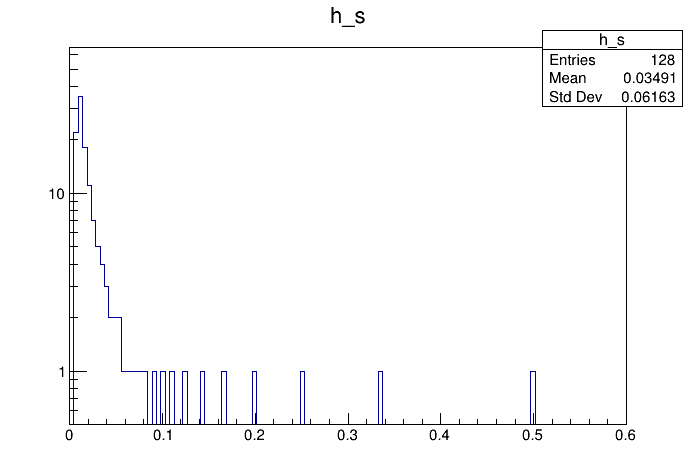# Df 0 1 0_Trivial Data Source¶

This tutorial illustrates how use the RDataFrame in combination with a RDataSource. In this case we use a TTrivialDS, which is nothing more than a simple generator: it does not interface to any existing dataset. The TTrivialDS has a single column, col0, which has value n for entry n.

Author: Danilo Piparo
This notebook tutorial was automatically generated with ROOTBOOK-izer from the macro found in the ROOT repository on Sunday, January 19, 2020 at 12:52 AM.

In :
import ROOT

Welcome to JupyROOT 6.19/01


Create the data frame

In :
MakeTrivialDataFrame = ROOT.RDF.MakeTrivialDataFrame

nEvents = 128

d_s = MakeTrivialDataFrame(nEvents)


Now we have a regular RDataFrame: the ingestion of data is delegated to the RDataSource. At this point everything works as before.

In :
h_s = d_s.Define("x", "1./(1. + col0)").Histo1D(("h_s", "h_s", 128, 0, .6), "x")

c = ROOT.TCanvas()
c.SetLogy()
h_s.Draw()


Draw all canvases

In :
from ROOT import gROOT
gROOT.GetListOfCanvases().Draw()